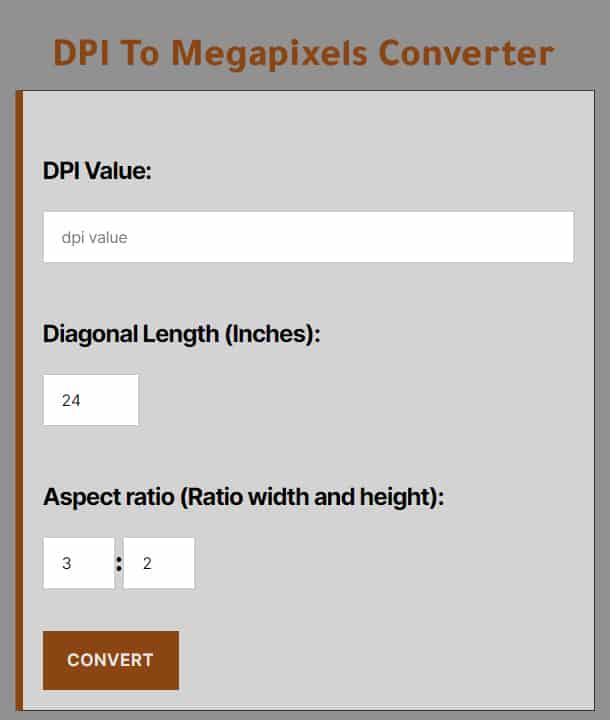# DPI To Megapixels Converter

#### DPI To Megapixels Converter

This is a free and accurate dpi to megapixels converter that you can use to convert dpi to megapixels easily. convert dpi to megapixels online perfectly.

##### Aspect ratio (Ratio width and height):

:

DPI is an abbreviation for “Dots Per Inch” and stands for the number of dots (pixels) per inch (image resolution).

The measure of the resolution of a monitor, printer, scanner, and other devices is determined by the number of DPI dots per inch. Such a measure is used to know the resolution of flat media

The smallest part of a digital image, a pixel, is a square or non-square image element, like a piece of a puzzle. A megapixel (MP) contains 1,000,000 pixels, and the number of megapixels offered by a camera system is the amount of information it can transfer into a digital photo or video.

#### How to use dpi to megapixels converter

First, enter the dpi value which you want to convert to megapixels

Second, enter the diagonal length in inches

Third, enter the aspect ratio

Finally, click on the convert button, that’s all!

#### DPI to megapixels conversion formula

To convert dpi to megapixels, you can use the above online converter for fast and one-click conversion, or you can use the dpi to megapixels conversion formula to manually calculate the result.

To manually convert dpi to megapixels, you need the following formulas:

First, calculate diagonal length in pixels using this formula:

Diagonal length (pixels) = DPI * diagonal length (inches)

Secondly, calculate height in pixels using the following formula:

Height (pixels) = Diagonal length (pixels)/√(Aspect ratio²+1)

Thirdly, calculate the width in pixels using the following formula:

Width (pixels) = Diagonal length (pixels)/√((1/Aspect ratio²)+1)

Finally, calculate the megapixels value using the following formula:

Megapixels (MP) = Height (pixels) * Width (pixels)

The best way to convert dpi to megapixels is using the above online converter because it is fast and easy to use.#### DPI to megapixels conversion chart

This is a chart for the common results of conversion dpi to megapixels at 3:2 aspect ratio and 24 inches diagonal length.

DPI Megapixels
72 DPI 1.38 Megapixels
150 DPI 5.98 Megapixels
200 DPI 10.63 Megapixels
250 DPI 16.62 Megapixels
300 DPI 23.93 Megapixels